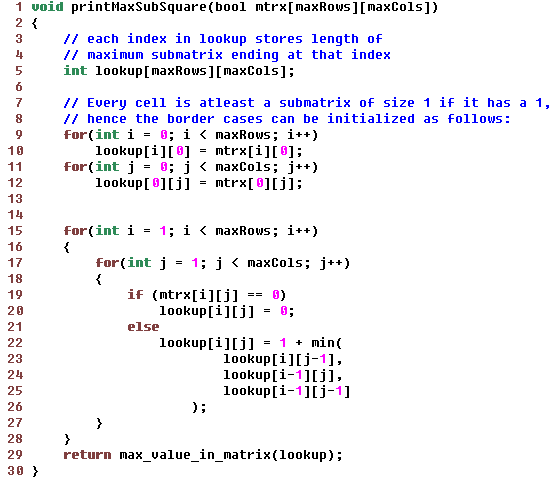Data Structuresand Algorithms Good OldJava AdvancedInterview Topics Cloud andDatabases Web Designand Development Must-knowTools Good ToKnow

## Find biggest sub-matrix which has only ones

Given a matrix composed of 1s and 0s only, find the biggest sub-matrix which is entirely composed of 1s only.

Solution: If there were only cell in the matrix, then biggest sub-matrix is that cell itself if it has a 1

Now, if you introduce an extra column, the biggest sub-matrix can be on length 2 if both of them are 1

i.e. F(2) = 1+F(1)

So, if we store the biggest sub-matrices ending at every index in the matrix, then addition of row or a column (at k+1th position) can just consider the ones at row k and column k to see if k+1 row or column will have bigger matrices.

Note: It is difficult to say which matrix is bigger if we consider non-square matrices also. Example: if 2 matrices have dimension, 4x4 and 3x5, both ending at index 5,5, then we would have to keep both of them because addition of 6th row/column can result in 3x6, 4x5 or 4x5, 5x5 matrices at index 6,6

To simplify, we will consider only those matrices which are square matrices i.e. K by K matrices only. With this simplification, every index can have maximum of only 1 sub-matrix

A lookup matrix can be made into which we will incrementally store maximum sub-matrices by adding one row/column at a time.The above figure explains the lookup relation:

lookup[i][j] = 1 + min (

lookup[i][j-1],

lookup[i-1][j],

lookup[i-1][j-1]

)

With the above observations, the code can be written as follows:Order for this solution is O(n2) or O(maxRows x maxCols)

Like us on Facebook to remain in touch
with the latest in technology and tutorials!

Got a thought to share or found a
bug in the code?
We'd love to hear from you:

 Name: Email: (Your email is not shared with anybody) Comment: